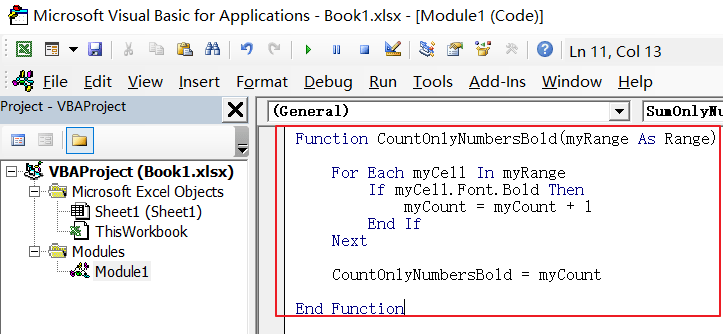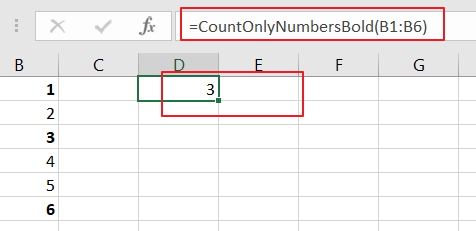# How to Count Only Numbers in Bold in a Range of Cells in Excel

This post will guide you how to Count only numbers with bold style in a range of cells in Excel. How do I Count on cells with bold font within a range of cells using User defined function in Excel 2013/2016.

## Count  Only Numbers with Bold Font Style

Assuming that you have a list of data in range B1:B6, which contain some bold or normal numbers. and you wish to Count only numbers with bold font style, how to quickly count those number based on bold font style in the given range of cells in Excel. And this tuotrial will show you how to count only the bold numbers with a User Defined Function in your worksheet. Just do the following steps:

Step1# open your excel workbook and then click on “Visual Basic” command under DEVELOPER Tab, or just press “ALT+F11” shortcut.Step2#  then the “Visual Basic Editor” window will appear.

Step3# click “Insert” ->”Module” to create a new module.Step4# paste the below VBA code into the code window. Then clicking “Save” button.```Function CountOnlyNumbersBold(myRange As Range)

For Each myCell In myRange
If myCell.Font.Bold Then
myCount = myCount + 1
End If
Next

CountOnlyNumbersBold = myCount

End Function```

Step5# back to the current worksheet, then type the following formula in a blank cell , and then press Enter key.

`=CountOnlyNumbersBold(B1:B6)`Related Posts

How to Extract First Letter from Each Word in a Cell in Excel

This post will guide you how to extract first letter from each word in a given cell in Excel. How do I extract the first letter of each word in a range of names in your current worksheet in Microsoft ...

How to ignore Blank Cells while Concatenating cells in Excel

This post will guide you how to concatenate cells but ignore all blank cells in your worksheet in Excel. How do I concatenate cells but ignore blank cells with a formula in Excel. How to create a concatenate formula to ...

How to Calculate the Average Excluding the Smallest & Highest Numbers in Excel

Calculating the average for a batch of data is frequently used in our daily life. But for some cases like statistic the average score in a competition, or price analysis, we often calculate the average excluding the smallest and highest ...

How to Get the Maximum or Minimum Absolute Value in Excel

It is easy to find the maximum or minimum value in a batch of data in excel, but if this batch of numbers contains both positive and negative numbers, the maximum or minimum absolute value cannot be found out by ...

How to Sum Only Numbers in Bold in a Range of Cells in Excel

This post will guide you how to sum only numbers with bold style in a range of cells in Excel. How do I sum on cells with bold font within a range of cells using User defined function in Excel ...

How to Filter Formula in Excel

This post will guide you how to filter your data with an Formula in Excel. How do I filter cells with formulas through a User defined function in Excel 2013/2016. Filter Formula Assuming that you have a list of data ...

How to Count Cells by Font Color in Excel

This post will guide you how to count cells by font color in Excel. How do I Count the number of cells with specific cell Font color by using a User Defined function in Excel. Count Cells by Font Color ...

How to Highlight Cells Containing Formulas in Excel

This post will guide you how to highlight cells in which contain formulas using Conditional Formatting feature in Excel. How do I conditionally format a cell if it contains formula using a User defined function in combination with Conditional Formatting ...

How to Extract Number from Text String in Excel

This post will guide you how to extract number from a given test string in Excel. How do I extract all numbers from string using a formula in Excel. How to get all number from a given test string using ...

How to Filter Data with Strikethrough Format in Excel

This post will guide you how to filter or delete rows with strikethrough format in your selected range of cells in Excel. How do I Filter by strikethrough formatted text with Find and Replace function in Excel 2013/2016. Or how ...

Sidebar